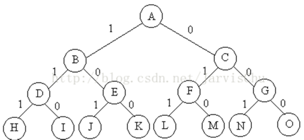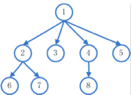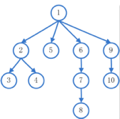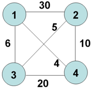# 五大常用算法学习笔记

1. 基本概念：把一个复杂的问题分成若干个相同或相似的子问题，再把子问题分成更小的子问题… ， 知道最后子问题可以简单的直接求解，原问题的解即子问题的解的合并。

1. 分治算法的适用场景：
1）当问题规模缩小到一定的程度就可以很容易解决
2）该问题可以分解为若干个规模较小的相同问题
3）该问题分解出的若干子问题的解可以合并为该问题的解
4）每个子问题都是独立的，相互之间没有交集。

2. 使用分治法的经典场景：
1）二分搜索
2）大整数乘法
3）合并排序
4）快速排序
5）汉诺塔

3. 分治算法经典例题：
输入一组整数，求出这组数字子序列和中最大值。也就是求出最大子序列的和，不必求出最大的那个序列。例如：序列：-2, 11, -1, 13, -5, -2 , 则最大子序列的和为20。

public static void main(String[] args) {
int[] a = { -2, 11, -4, 13, -5, -2 };// 最大子序列和为20
int[] b = { -6, 2, 4, -7, 5, 3, 2, -1, 6, -9, 10, -2 };// 最大子序列和为16
System.out.println(maxSubSum1(a));
System.out.println(maxSubSum4(b));
}

// 最大子序列求和算法一
public static int maxSubSum1(int[] a) {

int maxSum = 0;

// 从第i个开始找最大子序列和
for (int i = 0; i < a.length; i++) {

// 找第i到j的最大子序列和
for (int j = i; j < a.length; j++) {

int thisSum = 0;

// 计算从第i个开始，到第j个的和thisSum
for (int k = i; k <= j; k++) {
thisSum += a[k];
}
// 如果第i到第j个的和小于thisSum，则将thisSum赋值给maxSum
if (thisSum > maxSum) {
maxSum = thisSum;
}
}
}
return maxSum;
}

// 时间复杂度O(n的平方)
public static int maxSubSum2(int[] a) {
int maxSum = 0;
for (int i = 0; i < a.length; i++) {
// 将sumMax放在for循环外面，避免j的变化引起i到j的和sumMax要用for循环重新计算
int sumMax = 0;
for (int j = i; j < a.length; j++) {
sumMax += a[j];
if (sumMax > maxSum) {
maxSum = sumMax;
}
}
}
return maxSum;
}

// 递归，分治策略
// 2分logn，for循环n，时间复杂度O(nlogn)
public static int maxSubSum3(int[] a) {
return maxSumRec(a, 0, a.length - 1);
}

public static int maxSumRec(int[] a, int left, int right) {
// 递归中的基本情况
if (left == right) {
if (a[left] > 0)
return a[left];
else
return 0;
}
int center = (left + right) / 2;
// 最大子序列在左侧
int maxLeftSum = maxSumRec(a, left, center);
// 最大子序列在右侧
int maxRightSum = maxSumRec(a, center + 1, right);
// 最大子序列在中间（左边靠近中间的最大子序列+右边靠近中间的最大子序列）
int maxLeftBorderSum = 0, leftBorderSum = 0;
for (int i = center; i >= left; i--) {
leftBorderSum += a[i];
if (leftBorderSum > maxLeftBorderSum)
maxLeftBorderSum = leftBorderSum;
}
int maxRightBorderSum = 0, rightBorderSum = 0;
for (int i = center + 1; i <= right; i++) {
rightBorderSum += a[i];
if (rightBorderSum > maxRightBorderSum)
maxRightBorderSum = rightBorderSum;
}
// 返回最大子序列在左侧，在右侧，在中间求出的值中的最大的
return max3(maxLeftSum, maxRightSum, maxLeftBorderSum + maxRightBorderSum);
}

public static int max3(int a, int b, int c) {
return a > b ? (a > c ? a : c) : (b > c ? b : c);
}

// 时间复杂度:O(n); 任何a[i]为负时，均不可能作为最大子序列前缀；任何负的子序列不可能是最有子序列的前缀
public static int maxSubSum4(int[] a) {
int maxSum = 0, thisSum = 0;
for (int j = 0; j < a.length; j++) {
thisSum += a[j];
if (thisSum > maxSum)
maxSum = thisSum;
else if (thisSum < 0)
thisSum = 0;
}
return maxSum;
}


1. 基本概念：将待求解的问题分解为若干个子问题，按顺序求解子问题，前一问题的解，为后一问题的求解提供有用的信息。在求解任一子问题时，列出各种可能的局部解，通过决策保留那些有可能达到最优的局部解，丢弃其他局部解。依次解决各种子问题，最后一个子问题就是初始问题的解。
由于动态规划算法解决的问题是有重叠的子问题，为了减少重复计算，对每一个子问题只解一次，将其不同阶段的不同状态保存在一个二维数组中。

1. 动态规划的适用场景
1）最优化原理：该问题的最优解所包含的子问题的解也是最优的。
2）无后效性：某阶段状态一旦确定，就不受这个状态以后决策的影响。即某状态以后的过程不会影响以前的状态，只与当前状态有关。
3）有重叠子问题：即子问题之间不相互独立，一个子问题的解在下一阶段决策中可能被多次使用到。

2. 经典问题：
给定两个字符串str1和str2，返回两个字符串的最长公共子序列，例如：str1=“1A2C3D4B56",str2=“B1D23CA45B6A”,"123456"和"12C4B6"都是最长公共子序列，返回哪一个都行。

dp值的求法如下：
dp[i][j]的值比如和dp[i-1][j], dp[i][j-1]相关，结合下面的代码来看，实际上从第一行和第一列开始计算的，

public static void main(String[] args) {
String A = "1A2C3D4B56";
String B = "B1D23CA45B6A";
System.out.println(findLCS(A, A.length(), B, B.length()));
}

public static int findLCS(String A, int Alen, String B, int Blen) {
int dp[][] = new int[Alen+1][Blen+1];
for (int i = 0; i < Alen; i++) {
for (int j = 0; j < Blen; j++) {
if (A.charAt(i) == B.charAt(j)) {
dp[i + 1][j + 1] = dp[i][j] + 1;
} else {
dp[i + 1][j + 1] = Math.max(dp[i + 1][j], dp[i][j + 1]);
}
}
}
return dp[Alen][Blen];
}


1. 下面是一个可以试用贪心算法解的题目，贪心解的确不错，可惜不是最优解。

[背包问题]有一个背包，背包容量是M=150。有7个物品，物品可以分割成任意大小。

要求尽可能让装入背包中的物品总价值最大，但不能超过总容量。

物品 A B C D E F G

重量 35 30 60 50 40 10 25

价值 10 40 30 50 35 40 30

分析：
目标函数： ∑pi最大

1）根据贪心的策略，每次挑选价值最大的物品装入背包，得到的结果是否最优呢？
2）每次挑选重量最小的物品装入背包，是否能得到最优解？
3）每次选取单位重量价值最大的物品，成为解本地的策略？

1）选取价值最大者。

2）选取重量最小，反例与上述类似

3）选取单位重量价值最大的物品。反例：
W=30

2）贪心算法的经典案例：

1. 基本概念：回溯算法是一个类似枚举的搜索尝试过程，主要在搜索尝试过程中寻找问题的解，当发现已不满足求解条件时，就“回溯”返回，尝试别的路径。深度优先。

2. 回溯算法的实现：递归和迭代

1. 回溯算法的经典问题：0-1背包问题1. 基本概念

1）广度优先搜索：使用队列的先进先出2）深度优先搜索：2. 分支限界法的经典问题：旅行售货员问题

1）问题描述：某售货员要到若干城市去卖商品，已知各城市之间的路程。他要选定一条从驻地出发，经过每个城市一次，最后回到驻地的路线，使总的路程最小。2）问题理解：

3）关键思路：

Node{
int s; //结点深度，即当前走过了几个城市
int x[MAX_SIZE]; //保存走到这个结点时的路径信息
}
MiniHeap{
//保存所有结点并提供一些结点的操作
}

06-1234103-154188
06-104万+
02-19
01-201万+
12-16
05-082万+
09-051万+
10-044983
02-241万+
02-083万+
02-18203
02-273095
08-031352
12-253万+
03-299623
08-03735